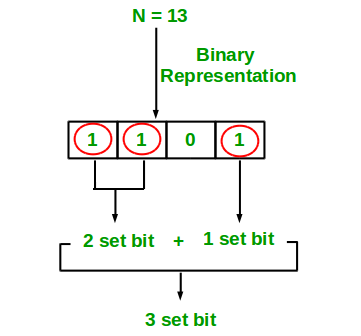# Java Program to Count set bits in an integer

• Last Updated : 02 Jan, 2019

Write an efficient program to count number of 1s in binary representation of an integer.

Examples :

```Input : n = 6
Output : 2
Binary representation of 6 is 110 and has 2 set bits

Input : n = 13
Output : 3
Binary representation of 11 is 1101 and has 3 set bits
```## Recommended: Please solve it on “PRACTICE ” first, before moving on to the solution.

1. Simple Method Loop through all bits in an integer, check if a bit is set and if it is then increment the set bit count. See below program.

## Java

 `// Java program to Count set``// bits in an integer``import` `java.io.*;`` ` `class` `countSetBits {``    ``/* Function to get no of set ``    ``bits in binary representation ``    ``of positive integer n */``    ``static` `int` `countSetBits(``int` `n)``    ``{``        ``int` `count = ``0``;``        ``while` `(n > ``0``) {``            ``count += n & ``1``;``            ``n >>= ``1``;``        ``}``        ``return` `count;``    ``}`` ` `    ``// driver program``    ``public` `static` `void` `main(String args[])``    ``{``        ``int` `i = ``9``;``        ``System.out.println(countSetBits(i));``    ``}``}`` ` `// This code is contributed by Anshika Goyal.`

Output:

```2
```

Recursive Approach :

## Java

 `// Java implementation of recursive``// approach to find the number``// of set bits in binary representation``// of positive integer n``import` `java.io.*;`` ` `class` `GFG {`` ` `    ``// recursive function to count set bits``    ``public` `static` `int` `countSetBits(``int` `n)``    ``{`` ` `        ``// base case``        ``if` `(n == ``0``)``            ``return` `0``;`` ` `        ``else`` ` `            ``// if last bit set add 1 else add 0``            ``return` `(n & ``1``) + countSetBits(n >> ``1``);``    ``}`` ` `    ``// Driver code``    ``public` `static` `void` `main(String[] args)``    ``{`` ` `        ``// get value from user``        ``int` `n = ``9``;`` ` `        ``// function calling``        ``System.out.println(countSetBits(n));``    ``}``}`` ` `// This code is contributes by sunnysingh`

Output:

```2
```

Please refer complete article on Count set bits in an integer for more details!

My Personal Notes arrow_drop_up